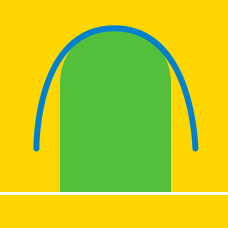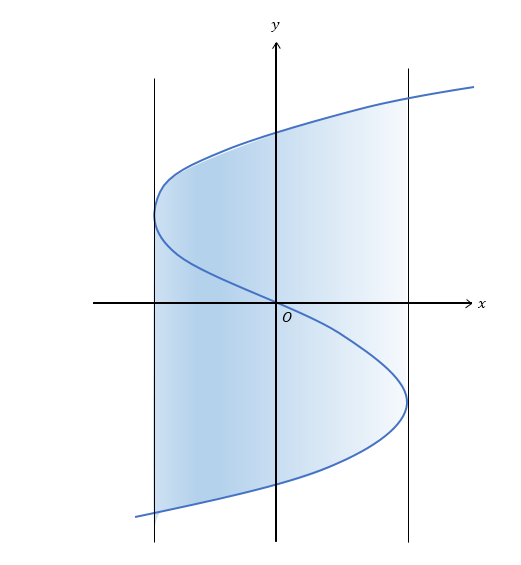Calculus

# Area between Curve and y-axisIf the blue curve in the above diagram represents the graph of $x={y}^3-300y$ and the vertical lines are tangent lines to the curve, what is the area of the shaded region?

What is the area of the region bounded by the curve $x= -{y}^2+100$ and the $y$-axis ?

Let $S$ be the area bounded by $y^2 \leq x$, $x \leq 25$ and $y \geq 0$. If $S = \frac{a}{b}$, where $a$ and $b$ are coprime positive integers, what is the value of $a+b$?

What is the area of the region bounded by the curve $x= -6y(y-4)$ and the $y$-axis ?

If $g(x)$ is the inverse function of $f(x)=10\cos x$ $(0 \leq x \leq \frac{\pi}{2}) ,$ what is the area of the region bounded by $y=g(x) ,$ the $x$-axis and $y$-axis?

×